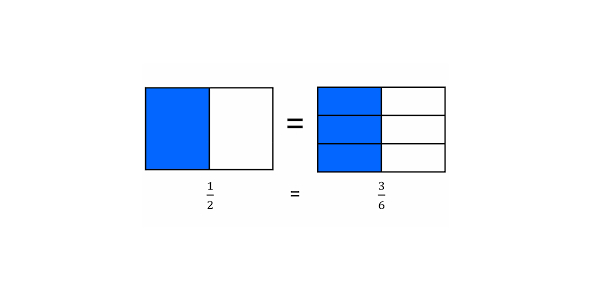# Equivalent Fractions Ultimate Quiz! Trivia

10 Questions | Total Attempts: 152SettingsWhat are equivalent fractions? Would you be willing to take this quiz? Equivalent fractions have the same value; even though they might look different, these fractions are the same. They have a different numerator and denominator but are equal to the same value. A fraction exemplifies a part of a whole or any number of equal parts. If you are exploring equivalent fractions, you have to attempt this great quiz.

• 1.
4 =    10  50
• 2.
2 = 1811
• 3.
2 = __5    20
• 4.
5 = ___12  60
• 5.
8 =  249
• 6.
3  =  ___4      100
• 7.
1  = ___9     63
• 8.
2  = ___3     18
• 9.
2  = ___8     56
• 10.
9  = 3612
Related TopicsBack to top